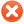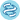Plot options# Use multiple genesUse list of genes Use mean expression of selected genes Use ratio of two genes Filter by median expression
more genes param

# ExampleExample input for multiple genes:
1007_s_at 1
200000_s_at -1
31637_s_at 0.5
40016_g_at -2

Explanation:
first: normal,
second: gene is inverted,
third: gene has weight,
fourth: gene has weight and is inverted

You can also use following format:
1007_s_at, 1
200000_s_at, -1
31637_s_at, 0.5
40016_g_at, -2

 Variant type: all mutations disruptive mutations coding mutations
 Survival: Overall survival (n=5997)Relapse free survival (n=3722)Follow up threshold: all2 months4 months6 months12 months18 months24 months30 months36 months48 months60 months120 months180 months240 monthsCensore at threshold:Select all (n=7874) Bladder Carcinoma (n=408) Breast cancer (n=980) Cervical squamous cell carcinoma (n=286) Colon adenocarcinoma (n=394) Cutaneous melanoma (n=465) Esophageal Squamous Cell Carcinoma (n=0) Head-neck squamous cell carcinoma (n=494) Kidney renal clear cell carcinoma (n=332) Kidney renal papillary cell carcinoma (n=278) Liver hepatocellular carcinoma (n=358) Low grade glioma (n=501) Lung adenocarcinoma (n=508) Lung squamous cell carcinoma (n=488) Ovarian cancer (n=272) Prostate adenocarcinoma (n=492) Sarcoma (n=235) Stomach adenocarcinoma (n=372) Thyroid carcinoma (n=485) Uterine corpus endometrial carcinoma (n=526)

## Restrict analysis to subtypes...

 Stage: all 1 (n=1549) 2 (n=1336) 3 (n=1051) 4 (n=593) Gender: all female (n=3619) male (n=2403) Race: all white (n=4337) asian (n=471) black/african american (n=643) Grade: all 1 (n=247) 2 (n=1028) 3 (n=1219) 4 (n=56) Mutation burden: all high (n=3049) low (n=2973)

## Restrict analysis based on cellular content…

 Basophils: all enriched (n=2746) decreased (n=2903) B-cells: all enriched (n=3114) decreased (n=2535) CD4+ memory T-cells: all enriched (n=3111) decreased (n=2538) CD8+ T-cells: all enriched (n=2809) decreased (n=2840) Eosinophils: all enriched (n=2437) decreased (n=3212) Macrophages: all enriched (n=3337) decreased (n=2312) Mesenchymal stem cells: all enriched (n=2763) decreased (n=2886) Natural killer T-cells: all enriched (n=2423) decreased (n=3226) Regulatory T-cells: all enriched (n=2854) decreased (n=2795) Type 1 T-helper cells: all enriched (n=2791) decreased (n=2858) Type 2 T-helper cells: all enriched (n=3331) decreased (n=2318)

Please note: the generated p value does not include correction for multiple hypothesis testing by default.n = number of patients with available clinical data

Please kindly cite our paper to support further development: Lanczky A, Gyorffy B. Web-Based Survival Analysis Tool Tailored for Medical Research (KMplot): Development and Implementation, J Med Internet Res, 2021; 23(7):e27633, doi:10.2196/27633